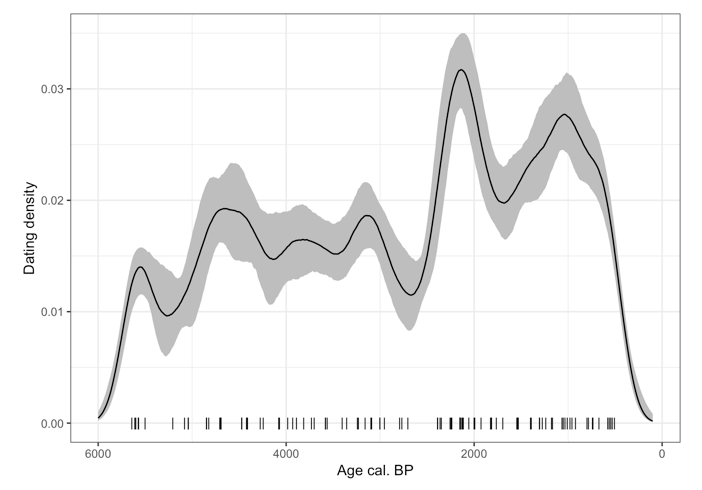Temporal density of 14C dating

In this tutorial I illustrate the methods that have been used to reconstruct the curve of the temporal density of archeological dating around the Folly peat bog in Blarquez et al. (2018).

The approach is inspired by ‘clam’ package age-depth modeling (Blaauw, 2010). For each archeological dating, we will randomly pick one age according to its calibrated probability density (i.e., ages with a higher probability are more likely to be selected). We will then use a kernel density function to assess the temporal density of archeological dating. We will repeat this procedure 1000 times to assess the 95% confidence interval (CI) around the median trend in archeological sites dating during the Holocene.

In the original paper the archeological 14C dates came from the CARD database for sites that were located within a 200 km radius of Folly peat bog (Martindale et al., 2016), and wer completed with an additional set of 7 dates from an archeological site in Gatineau, which had yet to be included in the database (Archeotec, 2014). In this example we will simply simulate some 14C dating:

# rm(list=ls())
library(Bchron)
library(ggplot2)

## Generate a random list of 14C dating --------------------------------------------------
set.seed(12)
age_14C=round(runif(100, 40, 500))*10
age_sd=round(runif(100, 2, 7))*10

We then calibrate each date in the database using the IntCal13 calibration curve (Reimer et al., 2013):

## Calibrate these dates using Bchron ---------------------------------------------------

age=BchronCalibrate(ages=age_14C,
ageSds=age_sd,
calCurves=rep('intcal13',length(age_14C)))

Here we define a custom function that will be used to sample an age within each dates distribution. This sampling is done according to the probability density distribution of each age. This procedure is somewhat equivalent with clam and bacon sampling procedure.

## Sample one age with probability (equivalent to clam and ?bacon) -----------------------
## Use lapply to sample inside a list of ages

foo=function(x){
y=sample(x\$ageGrid,1,prob=x\$densities)
return(y)
}
# Test a single iteration
ragelist=lapply(age,foo)
dates=as.vector(unlist(ragelist));dates

Now we will run this sampling 1000 times and for each iteration we will reconstruct the density of calibrated ages using kernel density estimation with a bandwidth of 150 years. We will subsequently calculate the median density of ages and 95% confidence intervals.

n=length(age_14C) #number of events

## Bootstrap --------------------------------------------------
nbboot=1000
fmat=matrix(nrow=length(age_),ncol=nbboot)

for(i in 1:nbboot){
ragelist=lapply(age,foo)
dates=as.vector(unlist(ragelist))
fmat[,i]=c(density(dates,
bw=150,kernel="gaussian",from=100,
to=6000, n=length(age_))\$y*n)
cat(i," ")
}

alpha=0.05
CI=apply(fmat, 1, quantile, probs=c(alpha/2,0.5,1-(alpha/2)) )

Plot the temporal trends in the density of archeological dating (along with max intercepts for each date sensu Telford et al. (2004), for display purpose only):

## Plot --------------------------------------------------------
bar=function(x){
z=x\$ageGrid[which.max(x\$densities)]
return(z)
}
magelist=lapply(age,bar)
mdates=as.vector(unlist(magelist));
df2=data.frame(x=mdates,y =0)

df=data.frame(age=age_, t(CI))
ggplot()+
geom_ribbon(data=df,aes(x=age,ymax=X97.5.,ymin=X2.5.),fill="grey")+
geom_line(data=df,aes(x=age_,y=X50.))+
geom_point(data=df2,aes(x,y),pch="|",size=3)+
scale_x_reverse()+
ylab("Dating density")+xlab("Age cal. BP")+
theme_bw()

## --------------------------------------------------------------

Archéotec, 2014. Travaux de réaménagement de la rue Jacques-Cartier, ville de Gatineau Site BiFw-172,

Blaauw, M., 2010. Methods and code for “classical” age-modelling of radiocarbon sequences. Quaternary Geochronology, 5(5), pp.512–518. Available at: http://dx.doi.org/10.1016/j.quageo.2010.01.002.

Blarquez, O. et al., 2018. Late Holocene influence of societies on the fire regime in southern Québec temperate forests. Quaternary Science Reviews, 180, pp.63–74. Available at: http://linkinghub.elsevier.com/retrieve/pii/S0277379117307060 [Accessed December 15, 2017].

Martindale, A. et al., 2016. Canadian Archaeological Radiocarbon Database (CARD 2.1), accessed 15 September 2017. , 2004.

Reimer, P.J. et al., 2013. IntCal13 and Marine13 Radiocarbon Age Calibration Curves 0–50,000 Years cal BP. Radiocarbon, 55(04), pp.1869–1887. Available at: https://www.cambridge.org/core/product/identifier/S0033822200048864/type/journal_article.

Telford, R.J., Heegaard, E. & Birks, H.J.B., 2004. The intercept is a poor estimate of a calibrated radiocarbon age. The Holocene, 14(2), pp.296–298. Available at: http://journals.sagepub.com/doi/10.1191/0959683604hl707fa.